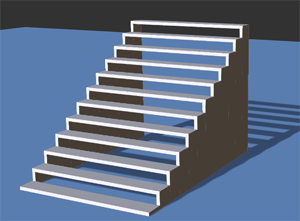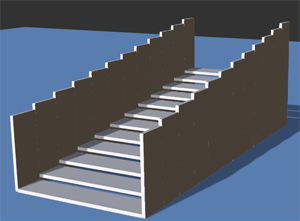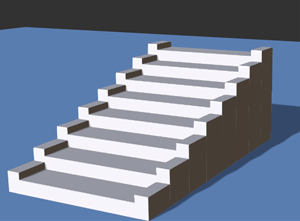# IC Python API:Linear Interpolation

Main article: Python of the Month.

This article will introduce the concept of linear interpolation and it's usefulness. This lesson will use linear interpolation to build a staircase and customize it with simple to understand attributes.

## Required Modules

Besides the rudimentary iClone Python module, we'll also be using os and winreg as a means to import a box prop to build the staircase.

```import RLPy
import os
import winreg
```

## Linear Interpoloation (Lerp) Formula

In mathematics, linear interpolation is a method of curve fitting using linear polynomials to construct new data points within the range of a discrete set of known data points. Simply put, linear interpolation can be used to retrieve the location of point along a line. Programmatically, we can reduce the complexity of this formula to simply give us a value between two given values based on far along the line we proceed.

```def lerp(value_a, value_b, interpolation):
# Precise method, which guarantees v = v1 when t = 1
return (1 - interpolation) * value_a + value_b * interpolation
```

The interpolation value is always normalized for the size of the line i.e. it is always a value clamped between 0 and 1.

## Creating a Box Prop

We'll need to create a box prop for constructing the staircase. This box prop will be used to construct the steps and the skirts (side panels) of the staircase.

```# Get the iClone 7 default template path
registry = winreg.ConnectRegistry(None, winreg.HKEY_LOCAL_MACHINE)
rawKey = winreg.OpenKey(registry, r"SOFTWARE\Reallusion\iClone\7.0")
ic_template_path = os.path.abspath(winreg.QueryValueEx(rawKey, "Template Data"))

# Load beveled Box.iProp and retrieve its control data block
box = RLPy.RScene.FindObject(RLPy.EObjectType_Prop, "Box")
box_control = box.GetControl("Transform")
box_db = box_control.GetDataBlock()
```

## Parameters

We can drive the construction of the staircase with a few attributes. These attributes can then be used to create a variety of staircases.

```height = 2 # Total height of the entire staircase
width = 2 # Total width of the entire staircase
depth = 3 # Total depth of the entire staircase
steps = 10 # the number of steps for the staircase
rail = 0.5 # Height of the rail beside the staircase
thickness = 0.05 # Thickness of each step
```

## Construction

Finally, we'll use a loop to create the steps the side skirts for each segment of the staircase.

```for x in range(0, steps):
t = x/steps

box_db.SetData("Position/PositionX", time, RLPy.RVariant(0))
box_db.SetData("Position/PositionY", time, RLPy.RVariant(lerp(0, depth * 100, t)))
box_db.SetData("Position/PositionZ", time, RLPy.RVariant(lerp(0, height * 100, t))) # Vertical
box_db.SetData("Scale/ScaleX", time, RLPy.RVariant(width)) # Width
box_db.SetData("Scale/ScaleY", time, RLPy.RVariant(depth / steps)) # Depth
box_db.SetData("Scale/ScaleZ", time, RLPy.RVariant(thickness)) # Height

step = box.Clone()
step.SetName("Step "+str(x+1))

box_db.SetData("Position/PositionX", time, RLPy.RVariant(width * 50))
box_db.SetData("Position/PositionZ", time, RLPy.RVariant(0)) # Vertical
box_db.SetData("Scale/ScaleX", time, RLPy.RVariant(thickness)) # Width
box_db.SetData("Scale/ScaleZ", time, RLPy.RVariant(lerp(thickness + rail, height + thickness + rail, t))) # Height

skirt = box.Clone()
skirt.SetName("Skirt_R "+str(x+1))

box_db.SetData("Position/PositionX", time, RLPy.RVariant(width * -50))

skirt = box.Clone()
skirt.SetName("Skirt_L "+str(x+1))

# Remove the initial prop, we don't need it anymore
RLPy.RScene.RemoveObject(box)
```

## Variations

We can create a diverse set of staircases by adjusting the parameters for construction.

•height: 2.2, width: 2, depth: 3, steps: 12, rail: 0, thickness: 0.05
•height: 1, width: 2, depth: 4, steps: 12, rail: 1, thickness: 0.05
•height: 1.5, width: 2, depth: 4, steps: 8, rail: 0.2, thickness: 0.2

## Everything Put Together

You can copy and paste the following code into a PY file and load it into iClone via Script > Load Python.

```import RLPy
import os
import winreg

def lerp(value_a, value_b, interpolation):
# Precise method, which guarantees v = v1 when t = 1
return (1 - interpolation) * value_a + value_b * interpolation

# Get the iClone 7 default template path
registry = winreg.ConnectRegistry(None, winreg.HKEY_LOCAL_MACHINE)
rawKey = winreg.OpenKey(registry, r"SOFTWARE\Reallusion\iClone\7.0")
ic_template_path = os.path.abspath(winreg.QueryValueEx(rawKey, "Template Data"))

# Load beveled Box.iProp and retrieve its control data block
box = RLPy.RScene.FindObject(RLPy.EObjectType_Prop, "Box")
box_control = box.GetControl("Transform")
box_db = box_control.GetDataBlock()

height = 2 # Total height of the entire staircase
width = 2 # Total width of the entire staircase
depth = 3 # Total depth of the entire staircase
steps = 10 # the number of steps for the staircase
rail = 0.5 # Height of the rail beside the staircase
thickness = 0.05 # Thickness of each step

time = RLPy.RTime(0)

for x in range(0, steps):
t = x/steps

box_db.SetData("Position/PositionX", time, RLPy.RVariant(0))
box_db.SetData("Position/PositionY", time, RLPy.RVariant(lerp(0, depth * 100, t)))
box_db.SetData("Position/PositionZ", time, RLPy.RVariant(lerp(0, height * 100, t))) # Vertical
box_db.SetData("Scale/ScaleX", time, RLPy.RVariant(width)) # Width
box_db.SetData("Scale/ScaleY", time, RLPy.RVariant(depth / steps)) # Depth
box_db.SetData("Scale/ScaleZ", time, RLPy.RVariant(thickness)) # Height

step = box.Clone()
step.SetName("Step "+str(x+1))

box_db.SetData("Position/PositionX", time, RLPy.RVariant(width * 50))
box_db.SetData("Position/PositionZ", time, RLPy.RVariant(0)) # Vertical
box_db.SetData("Scale/ScaleX", time, RLPy.RVariant(thickness)) # Width
box_db.SetData("Scale/ScaleZ", time, RLPy.RVariant(lerp(thickness + rail, height + thickness + rail, t))) # Height

skirt = box.Clone()
skirt.SetName("Skirt_R "+str(x+1))

box_db.SetData("Position/PositionX", time, RLPy.RVariant(width * -50))

skirt = box.Clone()
skirt.SetName("Skirt_L "+str(x+1))

# Remove the initial prop, we don't need it anymore
RLPy.RScene.RemoveObject(box)
```

### APIs Used

You can research the following references for the APIs deployed in this code.